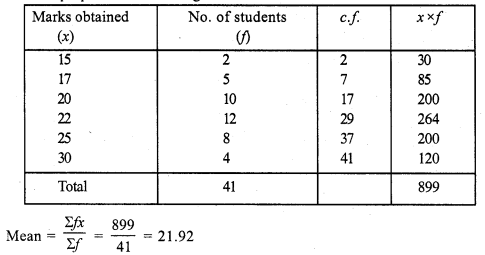# RS Aggarwal Class 7 Solutions Chapter 21 Collection and Organisation of Data Ex 21C

In this chapter, we provide RS Aggarwal Solutions for Class 7 Chapter 21 Collection and Organisation of Data Ex 21C for English medium students, Which will very helpful for every student in their exams. Students can download the latest RS Aggarwal Solutions for Class 7 Chapter 21 Collection and Organisation of Data Ex 21C Maths pdf, free RS Aggarwal Solutions Class 7 Chapter 21 Collection and Organisation of Data Ex 21C Maths book pdf download. Now you will get step by step solution to each question.

### RS Aggarwal Solutions for Class 7 Chapter 21 Collection and Organisation of Data Ex 21C Download PDF

Question 1.
Solution:
(i) Arranging in ascending order :
4, 6, 7, 8, 8, 8, 8, 10, 11, 15
We see that 8 occurs maximum times
Mode = 8
(ii) Arranging in ascending order :
18, 21, 23, 27, 27, 27, 27, 27, 36, 39, 40
We see that 27 occurs maximum times
Mode = 27

Question 2.
Solution:
Arranging in ascending order :
28, 31, 32, 32, 32, 32, 34, 36, 38, 40, 41.
We see that 32 occurs maximum times
Mode = 32 years

Question 3.
Solution:
We prepare the table as given below:Here, number of terms = 45, which is odd
Median = n+12 th term = 45+12 = 462 th term
= 23th term = 450
Now, mode = 3(median) – 2(mean)
= 3 x 450 – 2 x 470
= 1350 – 940
= 410

Question 4.
Solution:
We prepare the table as given below:Here, number of terms (N) = 41, which is odd
Median = n+12 th term = 41+12 th term
= 422 = 21 th term = 22 {value of 18 to 29 = 22}
Mode = 3 (median) – 2 (mean)
= 3 x 22 – 2 x 21.92 = 66 – 43.84 = 22.16

Question 5.
Solution:
We prepare the table as given below:All Chapter RS Aggarwal Solutions For Class 7 Maths

—————————————————————————–

All Subject NCERT Exemplar Problems Solutions For Class 7

All Subject NCERT Solutions For Class 7

*************************************************

I think you got complete solutions for this chapter. If You have any queries regarding this chapter, please comment on the below section our subject teacher will answer you. We tried our best to give complete solutions so you got good marks in your exam.

If these solutions have helped you, you can also share rsaggarwalsolutions.in to your friends.